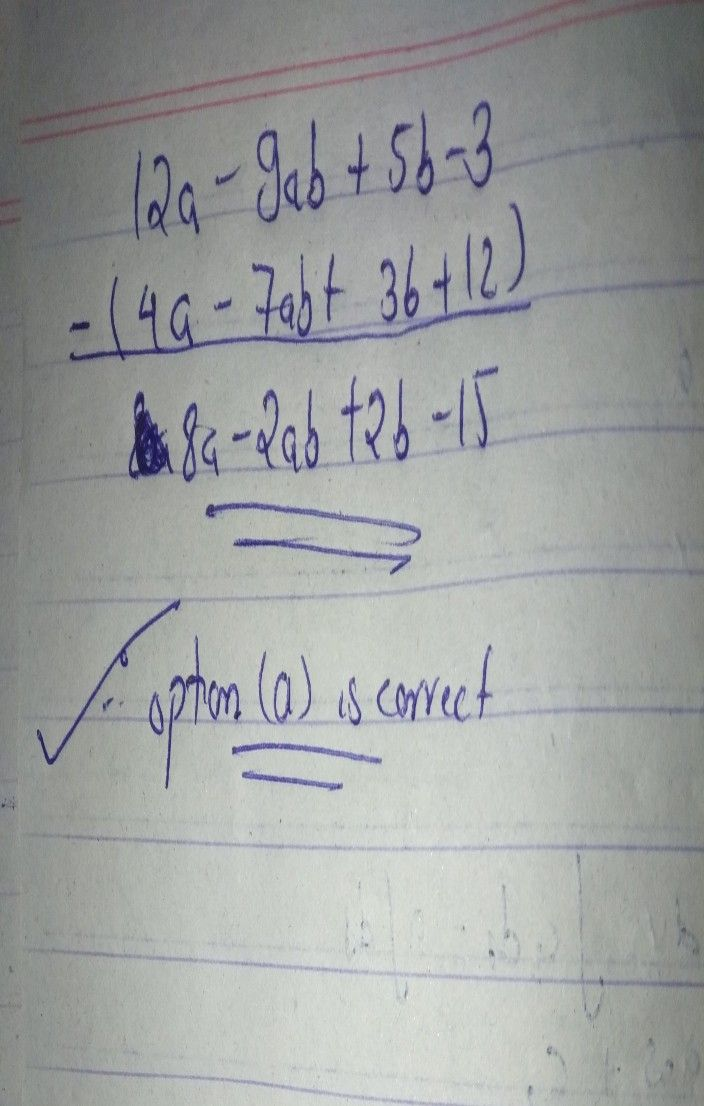Symbol
ProblemSubtract $4a-7ab+3b+12$ from $12a-$ $1$ point $9ab+5b-3$ $t$ $○$ $8a-2ab+2b-15$ $○$ $8a-2ab+2b+15$ $○$ $8a+2ab+2b-15$ $○$ $8a+2ab-2b+15$ Page $22$ of $102$
Algebra
SolutionQanda teacher - seena215Student
thank you! we appreciate your work! keep maintaining good quality of results:)